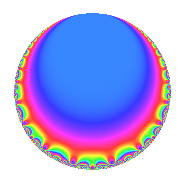# Properties

 Label 52.2.aLevel 52 Weight 2 Character orbit a Rep. character $$\chi_{52}(1,\cdot)$$ Character field $$\Q$$ Dimension 1 Newforms 1 Sturm bound 14 Trace bound 0

# Related objects

## Defining parameters

 Level: $$N$$ = $$52 = 2^{2} \cdot 13$$ Weight: $$k$$ = $$2$$ Character orbit: $$[\chi]$$ = 52.a (trivial) Character field: $$\Q$$ Newforms: $$1$$ Sturm bound: $$14$$ Trace bound: $$0$$

## Dimensions

The following table gives the dimensions of various subspaces of $$M_{2}(\Gamma_0(52))$$.

Total New Old
Modular forms 10 1 9
Cusp forms 5 1 4
Eisenstein series 5 0 5

The following table gives the dimensions of the cuspidal new subspaces with specified eigenvalues for the Atkin-Lehner operators and the Fricke involution.

$$2$$$$13$$FrickeDim.
$$-$$$$+$$$$-$$$$1$$
Plus space$$+$$$$0$$
Minus space$$-$$$$1$$

## Trace form

 $$q$$ $$\mathstrut +\mathstrut 2q^{5}$$ $$\mathstrut -\mathstrut 2q^{7}$$ $$\mathstrut -\mathstrut 3q^{9}$$ $$\mathstrut +\mathstrut O(q^{10})$$ $$q$$ $$\mathstrut +\mathstrut 2q^{5}$$ $$\mathstrut -\mathstrut 2q^{7}$$ $$\mathstrut -\mathstrut 3q^{9}$$ $$\mathstrut -\mathstrut 2q^{11}$$ $$\mathstrut -\mathstrut q^{13}$$ $$\mathstrut +\mathstrut 6q^{17}$$ $$\mathstrut -\mathstrut 6q^{19}$$ $$\mathstrut +\mathstrut 8q^{23}$$ $$\mathstrut -\mathstrut q^{25}$$ $$\mathstrut +\mathstrut 2q^{29}$$ $$\mathstrut +\mathstrut 10q^{31}$$ $$\mathstrut -\mathstrut 4q^{35}$$ $$\mathstrut -\mathstrut 6q^{37}$$ $$\mathstrut -\mathstrut 6q^{41}$$ $$\mathstrut +\mathstrut 4q^{43}$$ $$\mathstrut -\mathstrut 6q^{45}$$ $$\mathstrut -\mathstrut 2q^{47}$$ $$\mathstrut -\mathstrut 3q^{49}$$ $$\mathstrut +\mathstrut 6q^{53}$$ $$\mathstrut -\mathstrut 4q^{55}$$ $$\mathstrut -\mathstrut 10q^{59}$$ $$\mathstrut -\mathstrut 2q^{61}$$ $$\mathstrut +\mathstrut 6q^{63}$$ $$\mathstrut -\mathstrut 2q^{65}$$ $$\mathstrut +\mathstrut 10q^{67}$$ $$\mathstrut +\mathstrut 10q^{71}$$ $$\mathstrut +\mathstrut 2q^{73}$$ $$\mathstrut +\mathstrut 4q^{77}$$ $$\mathstrut -\mathstrut 4q^{79}$$ $$\mathstrut +\mathstrut 9q^{81}$$ $$\mathstrut -\mathstrut 6q^{83}$$ $$\mathstrut +\mathstrut 12q^{85}$$ $$\mathstrut -\mathstrut 6q^{89}$$ $$\mathstrut +\mathstrut 2q^{91}$$ $$\mathstrut -\mathstrut 12q^{95}$$ $$\mathstrut +\mathstrut 2q^{97}$$ $$\mathstrut +\mathstrut 6q^{99}$$ $$\mathstrut +\mathstrut O(q^{100})$$

## Decomposition of $$S_{2}^{\mathrm{new}}(\Gamma_0(52))$$ into irreducible Hecke orbits

Label Dim. $$A$$ Field CM Traces A-L signs $q$-expansion
$$a_2$$ $$a_3$$ $$a_5$$ $$a_7$$ 2 13
52.2.a.a $$1$$ $$0.415$$ $$\Q$$ None $$0$$ $$0$$ $$2$$ $$-2$$ $$-$$ $$+$$ $$q+2q^{5}-2q^{7}-3q^{9}-2q^{11}-q^{13}+\cdots$$

## Decomposition of $$S_{2}^{\mathrm{old}}(\Gamma_0(52))$$ into lower level spaces

$$S_{2}^{\mathrm{old}}(\Gamma_0(52)) \cong$$ $$S_{2}^{\mathrm{new}}(\Gamma_0(26))$$$$^{\oplus 2}$$